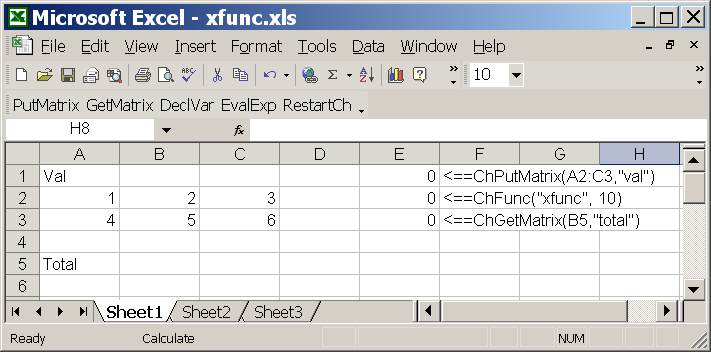SoftIntegration Demos for Ch and Toolkits
 Demos Ch Toolkit Demos Ch Mechanism Ch Control System Ch CGI Ch ODBC ChExcel C++ Library Demos Graphical Library Package Demos Ch NAG Statistics

ChExcel Demos.

All examples described in ChExcel User's Guide are included in the distribution of ChExcel and are readily to run once ChExcel is installed.

As an example, Excel spreadsheet xfunc.xls and function file xfunc.chf for function xfunc() described below are included in the distribution of ChExcel. The values in certain cells of the Excel spreadsheet xfunc.xls are passed to C function xfunc() for processing and the result from the function is then placed in a cell.

The program below defines a function xfunc() in function file xfunc.chf. The program begins by including the header file numeric.h with function prototype for numerical function sum(), followed by function prototype for xfunc() and declaration of the global variable total. The function xfunc() takes an integer as an argument, assigns the sum for each element of the array val declared in ChExcel to total, and returns the product n*total.

#include <numeric.h> double xfunc(int n); /* variable val is declared in ChExcel */ //array double val; /* declare global variable to be retrieved by ChExcel functions */ double total; double xfunc(int n) { total = sum(val); return n*total; } The first figure below shows the spreadsheet xfunc.xls. Type F2 from top to the bottom for 3 times by placing the cursor on "0" at the left below. 0 <==ChPutMatrix(A2:C3,"val") 0 <==ChFunc("xfunc",10) 0 <==ChGetMatrix(B5,"total")

Cell E1 uses ChExcel function ChPutMatrix() to declare a variable val and place the matrix in A2 through C3 into the variable val. Cell E2 uses ChExcel function ChFunc() to call the Ch function xfunc() with the argument 10. The returned value from calling function xfunc() in function file xfunc.chf is placed in Cell E2. Cell E3 uses ChExcel function ChGetMatrix() to get the value of variable total in Ch and places it in Cell B5. The resulting spreadsheet is shown in the second figure below.

The Excel spreadsheet before calling function xfunc().The Excel spreadsheet after calling function xfunc().